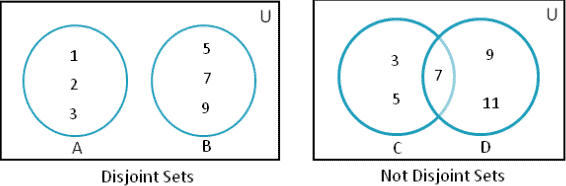# Python Set isdisjoint() Method: Complete Guide

Python Set isdisjoint() function checks whether the two sets are disjoint. If the sets are disjoint, then the isdisjoint() method returns True else, it returns False.

For example, if A={1,2,3} and B={5,7,9} are two sets, we can see that there is no common element in them, so they are known as disjoint.

See the following figure.## Python Set isdisjoint()

Python set isdisjoint() is a built-in function that tells us whether two are disjoint or not. The two sets are disjoint if they do not have any common elements.

Before learning the isdisjoint() method, we should learn what is Disjoint. Two sets are known to be disjoint if they do not have any shared values.

### Syntax

`set1.isdisjoint(set2)`

### Arguments

Here set1 is one Set, and set2 is another set.

### Return Value

Python isdisjoint() method returns two values, True if those sets are disjoint, False if given sets are not disjoint.

### Example

See the following code example.

```# app.py

# Declaring two sets
# Even nums between 2 and 10
set1 = {2, 4, 6, 8, 10}
# Multiple of 3 between 1 to 10
set2 = {3, 6, 9}
# Prime numbers from 1 to 10
set3 = {1, 3, 5, 7}

# priting both the sets
print("Set1 is: ", set1)
print("Set2 is : ", set2)
print("Set3 is: ", set3)
# Now we will find if they are disjoint
print("Are Set1 and Set2 disjoint? ", set1.isdisjoint(set2))
print("Are Set1 and Set3 disjoint? ", set1.isdisjoint(set3))
```

#### Output

```Set1 is:  {2, 4, 6, 8, 10}
Set2 is :  {9, 3, 6}
Set3 is:  {1, 3, 5, 7}
Are Set1 and Set2 disjoint?  False
Are Set1 and Set3 disjoint?  True
```

From the output, we can see that there is a common value between Set 1 and Set2 that is 6, so they are not disjoint. That’s why the result is False.

Also, we can see that there are no common elements in Set1 and Set3, so they are disjoint, and the result is True.

## Python Set isdisjoint() method with other iterables

When we pass the other iterables such as Listtuple, and dictionary in the isdisjoint() function, it converts them into the Set and then checks whether the sets are disjoint or not.

When converting the dictionary to Set, the keys are considered as the items of the converted Set.

See the following code example.

```# app.py

# Set A
A = {11, 21}

# List lis
lis = [11, 21, 46]

# Tuple t
t = (10, "Ankit")

# Dictionary dict, Set is formed on Keys
dict = {11: 'Eleven', 19: 'KK'}

# Dictionary dict2
dict2 = {'Four': 4, 'Five': 5}

print("Set A and List lis disjoint?", A.isdisjoint(lis))
print("Set A and tuple t are disjoint?", A.isdisjoint(t))
print("Set A and dict are disjoint?", A.isdisjoint(dict))
print("Set A and dict2 are disjoint?", A.isdisjoint(dict2))
```

#### Output

```python3 app.py
Set A and List lis disjoint? False
Set A and tuple t are disjoint? True
Set A and dict are disjoint? False
Set A and dict2 are disjoint? True```

So, two sets are said to be disjoint when their intersection is null. In simple words, they do not have any common element between them.

Finally, Python Set isdisjoint() Method Example is over.

Python set intersection_update()

Python set intersection()